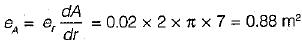Courses

# Test: Fundamental Concepts, Accuracy & Errors - 2

## 10 Questions MCQ Test GATE Civil Engineering (CE) 2022 Mock Test Series | Test: Fundamental Concepts, Accuracy & Errors - 2

Description
This mock test of Test: Fundamental Concepts, Accuracy & Errors - 2 for Civil Engineering (CE) helps you for every Civil Engineering (CE) entrance exam. This contains 10 Multiple Choice Questions for Civil Engineering (CE) Test: Fundamental Concepts, Accuracy & Errors - 2 (mcq) to study with solutions a complete question bank. The solved questions answers in this Test: Fundamental Concepts, Accuracy & Errors - 2 quiz give you a good mix of easy questions and tough questions. Civil Engineering (CE) students definitely take this Test: Fundamental Concepts, Accuracy & Errors - 2 exercise for a better result in the exam. You can find other Test: Fundamental Concepts, Accuracy & Errors - 2 extra questions, long questions & short questions for Civil Engineering (CE) on EduRev as well by searching above.
QUESTION: 1

### A circle of radius 7 m has a standard error of 0.02 m on the radius. The standard error of its area is

Solution:

Area of circle
A = πr2
∴ dA/dr = 2πr
Let standard error in radius be er,
∴ Standard error in area,QUESTION: 2

Solution:
QUESTION: 3

### The difference between the most probable value of a quantity and its observer value is

Solution:

True Error = Actual Value-Observed Value
Weighted Observations = Observations taken with proportion to any quantity like an area
Conditional Error = Error occurring due to certain conditions prevailing. Like temperature error.
Residual Error = Most Probable Value- Observed Value

QUESTION: 4

The error due to bad ranging is

Solution:

Cumulative Errors occurring in same direction and thus, they aggregate to make a considerable amount of error.
Positive cumulative errors Non Horizontality, sag, bad ranging.
Positive/ Negative cumulative errors Erroneous length of chain, temperature variation.

QUESTION: 5

For a well-conditional triangle, no angle should be less than

Solution:

For a well-conditioned triangle all the angles must lie in the range of 300-1200.

QUESTION: 6

An invar tape is made of an alloy of

Solution:
QUESTION: 7

Offsets are

Solution:

The distance measured right or left of the chain line to locate details like boundaries, culverts etc. are called offsets.
These are of two types:
(i) Perpendicular offsets at right angles to the chain line
(ii) oblique offsets not at right angles to the chain line

QUESTION: 8

‘Ranging' is the process of

Solution:
QUESTION: 9

Theory of probability is applied to

Solution:
QUESTION: 10

Systematic errors are those errors

Solution: